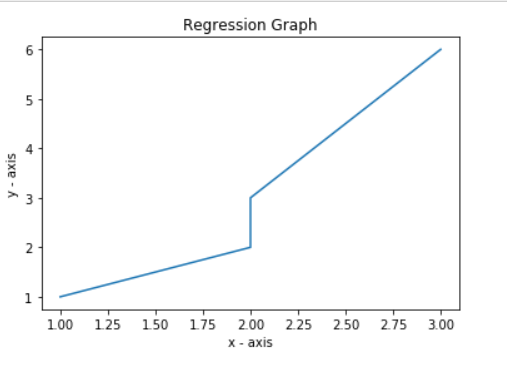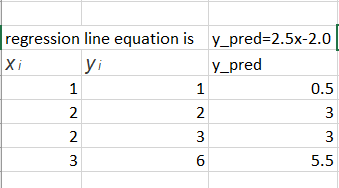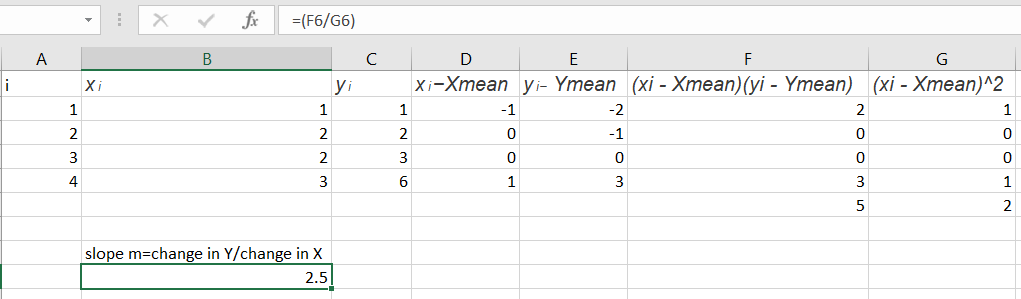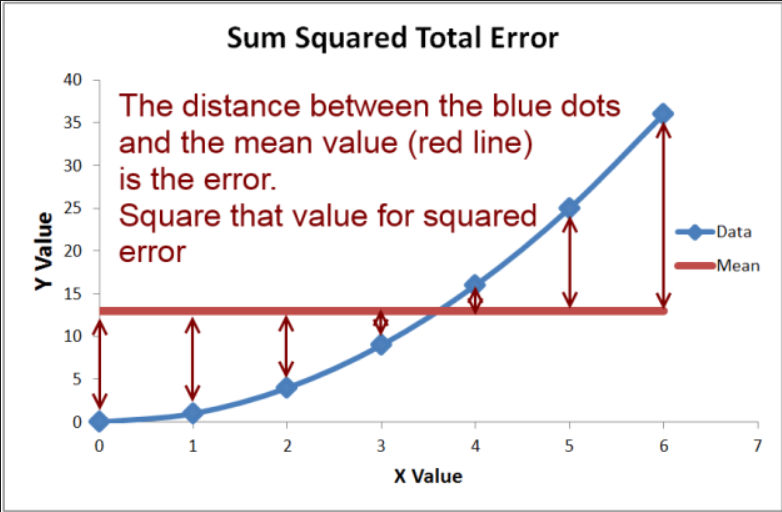Related Articles

# ML | Mathematical explanation of RMSE and R-squared error

• Last Updated : 02 Aug, 2019

RMSE: Root Mean Square Error is the measure of how well a regression line fits the data points. RMSE can also be construed as Standard Deviation in the residuals.
Consider the given data points: (1, 1), (2, 2), (2, 3), (3, 6).
Lets break the above data points into 1-d lists.
Input :

```x = [1, 2, 2, 3]
y = [1, 2, 3, 6]```

Code : Regression Graph

 `import` `matplotlib.pyplot as plt ``import` `math`` ` `# plotting the points  ``plt.plot(x, y) `` ` `# naming the x axis ``plt.xlabel(``'x - axis'``) `` ` `# naming the y axis ``plt.ylabel(``'y - axis'``) `` ` `# giving a title to my graph ``plt.title(``'Regression Graph'``) `` ` `# function to show the plot ``plt.show() `Code: Mean Calculation

 `# in the next step we will find the equation of the best fit line``# we will use Linear algebra's Point slope form to find regression line equation``# point-slope form is represented by y = mx + c``# where m is slope means (change in y) / (change in x)``# c is constant, it represents at which point line will intercept y-axis``# slope m can be formulated as below:``'''``   ``n``m =? (xi - Xmean) (yi - Ymean)/?(xi - Xmean)^2`` ``i = 1``'''``# calculate Xmean and Ymean``ct ``=` `len``(x)``sum_x ``=` `0``sum_y ``=` `0`` ` `for` `i ``in` `x:``    ``sum_x ``=` `sum_x ``+` `i``x_mean ``=` `sum_x ``/` `ct``print``(``'Value of X mean'``, x_mean)`` ` `for` `i ``in` `y:``    ``sum_y ``=` `sum_y ``+` `i``y_mean ``=` `sum_y ``/` `ct``print``(``'value of Y mean'``, y_mean)`` ` `# we have the values of x mean and y_mean`

Output :

```Value of X mean 2.0
value of Y mean 3.0
```

Code : Line Equation

 `# below is the process of finding line equation in mathematical terms``# slope of our line is 2.5``# calculate c to find out the equation`` ` `m ``=` `2.5``c ``=` `y_mean ``-` `m ``*` `x_mean``print``(``'Intercept'``, c)`

Output :

`Intercept -2.0`

Code : Mean Squared Error

 `# equation of our Regression line comes out to be as below:``# y_pred = 2.5x-2.0``# we call the line y_pred``# paste regression line graph``from` `sklearn.metrics ``import` `mean_squared_error ``# y_pred for our exusting data points is as below`` ` `y ``=``[``1``, ``2``, ``3``, ``6``]``y_pred ``=``[``0.5``, ``3``, ``3``, ``5.5``]``# root mean square calculated by sklearn package``mse ``=` `math.sqrt(mean_squared_error(y, y_pred))``print``(``'Root mean square error'``, mse)`

Output :

```Root mean square error 0.6123724356957945
```

Code : RMSE Calculation

 `# lets check how the Root mean square is calculated mathematically``# lets introduce a term called residuals``# residual are basically the distance of data point from the regression line``# residuals are denoted by red marked line in below graph``# root mean square and residuals are calculated as below``# we have 4 data points ``'''``r = 1, ri = yi-y_pred``y_pred is mx + c``ri = yi-(mx + c) ``e.g. x = 1, we have value of y as 1``we want to evaluate what exactly our model has predicted for x = 1``(1, 1)r1 = 1, x = 2 ``'''``# y_pred1 = 1-(2.5 * 1-2.0)= 0.5``r1 ``=` `1``-``(``2.5` `*` `1``-``2.0``)`` ` `#(2, 2) r2 = 2, x = 2 ``# y_pred2 = 2-(2.5 * 2-2.0)=-1``r2 ``=` `2``-``(``2.5` `*` `2``-``2.0``)`` ` `#(2, 3) r3 = 3, x = 2 ``# y_pred3 = 3-(2.5 * 2-2.0)= 0``r3 ``=` `3``-``(``2.5` `*` `2``-``2.0``)`` ` `#(3, 6) r4 = 4, x = 3 ``# y_pred4 = 6-(2.5 * 3-2.0)=.5``r4 ``=` `6``-``(``2.5` `*` `3``-``2.0``)`` ` `# from above calculation we have values of residuals``residuals ``=``[``0.5``, ``-``1``, ``0``, .``5``]`` ` `# now calculate root mean square error``# N = 4 data points``N ``=` `4``rmse ``=` `math.sqrt((r1``*``*``2` `+` `r2``*``*``2` `+` `r3``*``*``2` `+` `r4``*``*``2``)``/``N)``print``(``'Root Mean square error using maths'``, rmse)`` ` `# root mean square actually calculated using mathematics``# both of RMSE calculated are same`Output :

`Root Mean square error using maths 0.6123724356957945`

R-squared Error or Coefficient of Determination
R2 error answers the below question.
How much y varies with variation in x.Basically the % variation of y on variation with xCode : R-Squared Error

 `# SEline =(y1-(mx1 + b)**2 + y2-(mx2 + b)**2...+yn-(mxn + b)**2)``# SE_line =(1-(2.5 * 1+(-2))**2 + (2-(2.5 * 2+(-2))**2) +(3-(2.5*(2)+(-2))**2) + (6-(2.5*(3)+(-2))**2))`` ` `val1 ``=``(``1``-``(``2.5` `*` `1``+``(``-``2``)))``*``*``2``val2 ``=``(``2``-``(``2.5` `*` `2``+``(``-``2``)))``*``*``2``val3 ``=``(``3``-``(``2.5` `*` `2``+``(``-``2``)))``*``*``2``val4 ``=``(``6``-``(``2.5` `*` `3``+``(``-``2``)))``*``*``2``SE_line ``=` `val1 ``+` `val2 ``+` `val3 ``+` `val4``print``(``'val'``, val1, val2, val3, val4)`` ` `# next to calculate total variation in Y from mean value``# variation in y is calcualted as``# y_var =(y1-ymean)**2+(y2-ymean)**2...+(yn-ymean)2`` ` `y ``=``[``1``, ``2``, ``3``, ``6``]`` ` `y_var ``=``(``1``-``3``)``*``*``2``+``(``2``-``3``)``*``*``2``+``(``3``-``3``)``*``*``2``+``(``6``-``3``)``*``*``2``SE_mean ``=` `y_var`` ` `# by calculating y_var we are calculating the distance``# between y data points and mean value of y``# so answer to our question, % of the total variation``# of wrt x is denoted as below:``r_squared ``=` `1``-``(SE_line ``/` `SE_mean)`` ` `# [SE_line / SE_mean] -->tells us the what % of variation``# in y is not described by regression line``# 1-(SE_line / SE_mean) --> gives us the exact value of ``# how much % y varies with variation in x``print``(``'Rsquared error'``, r_squared)`

Output :

```Rsquared error 0.8928571428571429
```

Code : R-Squared Error with sklearn

 `from` `sklearn.metrics ``import` `r2_score`` ` `# r2 error calculated by sklearn is similar ``# to ours mathematically calculated r2 error``# calculate r2 error using sklearn``r2_score(y, y_pred)`

Output :

`0.8928571428571429`

Attention reader! Don’t stop learning now. Get hold of all the important Machine Learning Concepts with the Machine Learning Foundation Course at a student-friendly price and become industry ready.

My Personal Notes arrow_drop_up